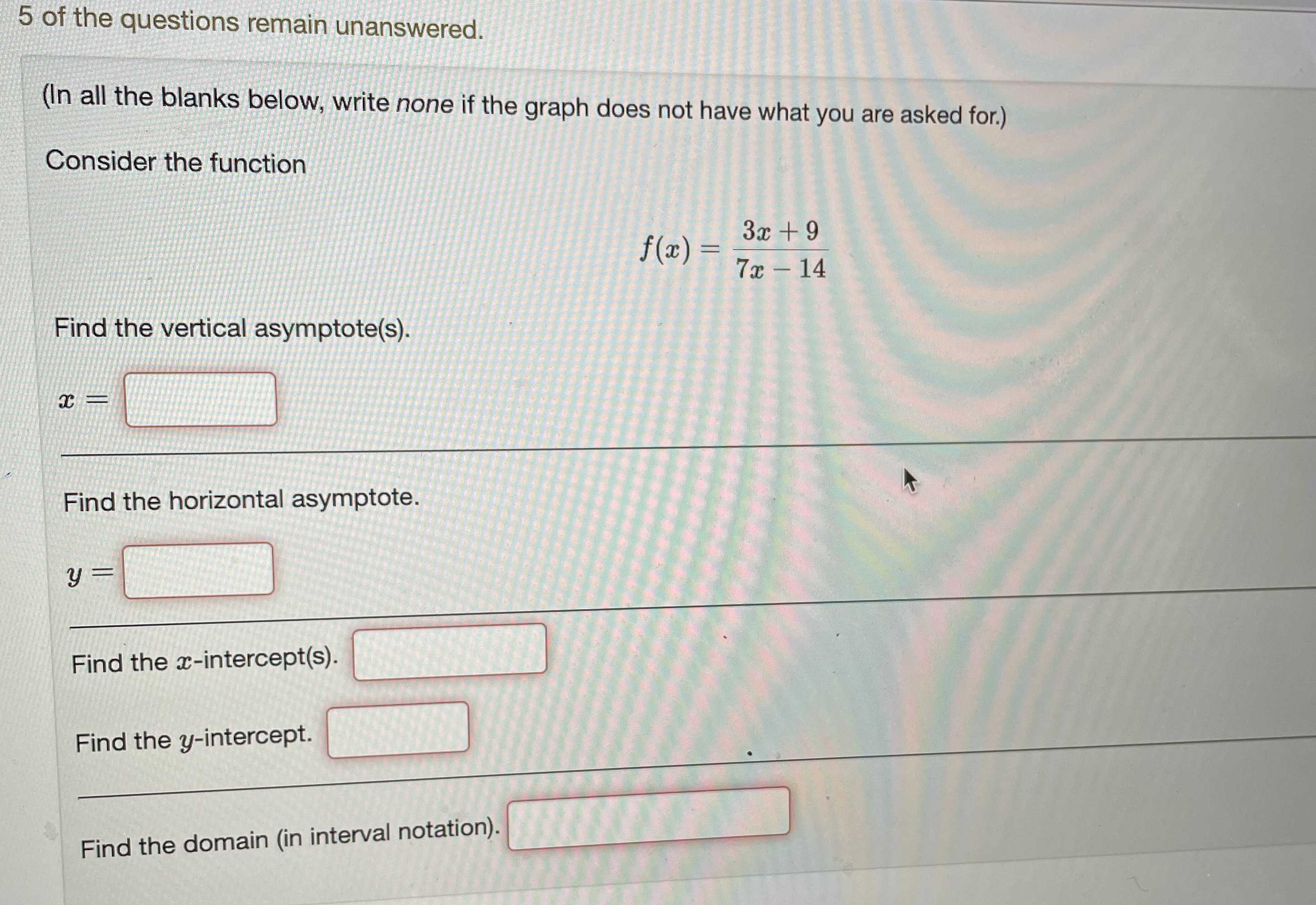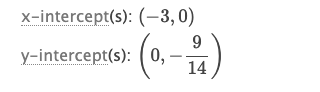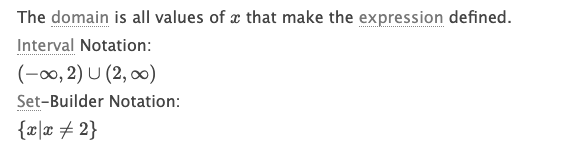### ¿Todavía tienes preguntas de matemáticas?

Pregunte a nuestros tutores expertos
Algebra
Pregunta$$5$$ of the questions remain unanswered. (In all the blanks below, write none if the graph does not have what you are asked for.) Consider the function Find the vertical asymptote(s).

$$x = \frac { 3 x + 9 } { 7 x - 14 }$$

Find the horizontal asymptote.

$$y =$$